Verify Generated Code by Using Cosimulation

Verify your generated code by using cosimulation. The generated code and testbench run on a Codesys software-based programable logic controller (soft PLC). The Simulink ™ model uses Open Platform Communication Unified Architecture (OPC UA) to communicate with and retrieve the cosimulated data from the soft PLC. The Simulink model verifies the generated code by comparing the model simulation results to the cosimulated soft PLC results.

Prerequisites

On your system, you must have installed:

• Codesys V3.5.SP16 + IDE or Codesys V3.5 SP16 Patch 1 + IDE

• OPC Toolbox ™

Model Description

The simple_cosim model is made up of the SimpleSubsystem block and the MATLAB System (PLCCOSIM) block. During simulation, the model compares values from the SimpleSubsystem block to the values from the soft plc which are retrieved by using the MATLAB System (PLCCOSIM) block.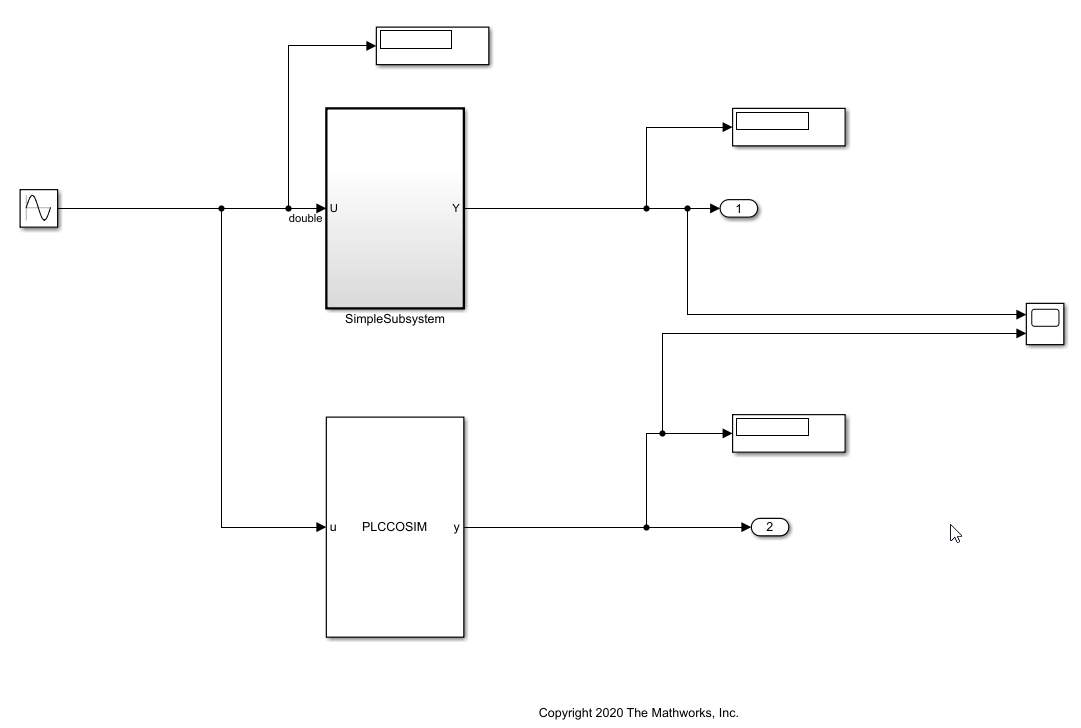The SimpleSubsystem block contains a simple feedback loop.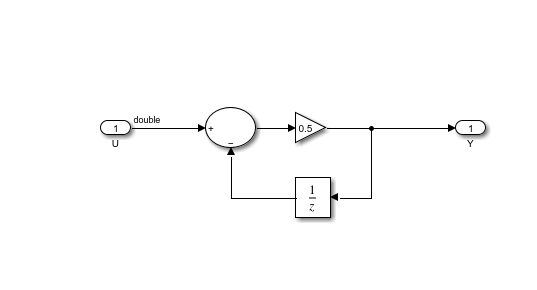The MATLAB System block is set up to run the PLCCOSIM.m file. The file contains the code to set up OPC UA communications and retrieve the cosimulated data from the soft PLC.

type PLCCOSIM.m
classdef PLCCOSIM < matlab.System
%
% This template includes the minimum set of functions required
% to define a System object with discrete state.

% Public, tunable properties
properties

end

properties(DiscreteState)
CycleNum;
end

% Pre-computed constants
properties(Access = private)
UAObj;
DeviceNode;
Cycle_U;
Cycle_Y;
TestCycleNum;
PreviousCycleNum;
end

methods(Access = protected)
function setupImpl(obj)
% Perform one-time calculations, such as computing constants
% init opc UA server connection
obj.UAObj = opcua('opc.tcp://localhost:4840');
connect(obj.UAObj);
obj.DeviceNode = findNodeByName(obj.UAObj.Namespace,'DeviceSet','-once');
obj.Cycle_U = findNodeByName(obj.DeviceNode,'cycle_U');
obj.Cycle_Y = findNodeByName(obj.DeviceNode,'cycle_Y');
obj.TestCycleNum = findNodeByName(obj.DeviceNode,'testCycleNum');
obj.PreviousCycleNum = findNodeByName(obj.DeviceNode,'previousCycleNum');
end

function y = stepImpl(obj,u)
% Implement algorithm. Calculate y as a function of input u and
% discrete states.
obj.CycleNum = obj.CycleNum+1;
writeValue(obj.UAObj, obj.Cycle_U, u);
writeValue(obj.UAObj, obj.TestCycleNum, obj.CycleNum);

valueUpdated = false;
for rct = 1:100
if previousCycleNumValue == obj.CycleNum
valueUpdated = true;
break
end
pause(0.001)
end

if ~valueUpdated
error('not get the value for cycle number %d', obj.CycleNum);
end
end

function resetImpl(obj)
% Initialize / reset discrete-state properties
obj.CycleNum = 0;
writeValue(obj.UAObj, obj.TestCycleNum, obj.CycleNum);
writeValue(obj.UAObj, obj.PreviousCycleNum, obj.CycleNum);
end

function out = getOutputSizeImpl(obj)
out = propagatedInputSize(obj,1);
end

function out = getOutputDataTypeImpl(obj)
out = propagatedInputDataType(obj,1);
end

function c1 = isOutputComplexImpl(obj)
c1 = false;
end

function c1 = isOutputFixedSizeImpl(obj)
c1 = true;
end

function s = getDiscreteStateImpl(obj)
s = obj.CycleNum;
end
end
end

Compile, and Simulate the Codesys Project

The Codesys project file runs the generated code on the soft PLC. If you have the Codesys V3.5.SP16 + IDE, use the plc-cosim_v3sp16.project as the project file. If you have the Codesys V3.5.SP16 Patch 1+ IDE, use the plc-cosim_v3sp16patch1.project as the project file.

1. In the Windows ® system tray, select CODESYS Control Win SysTray -x64, right-click, and select Start PLC.

2. Open the project file based on the Codesys Target IDE version.

3. Double-click CODESYS_Control_Win_V3_x64 and select Scan Network.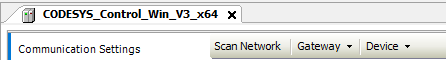4. In the Scan Network tab, select your device in the Select Device window. Click OK.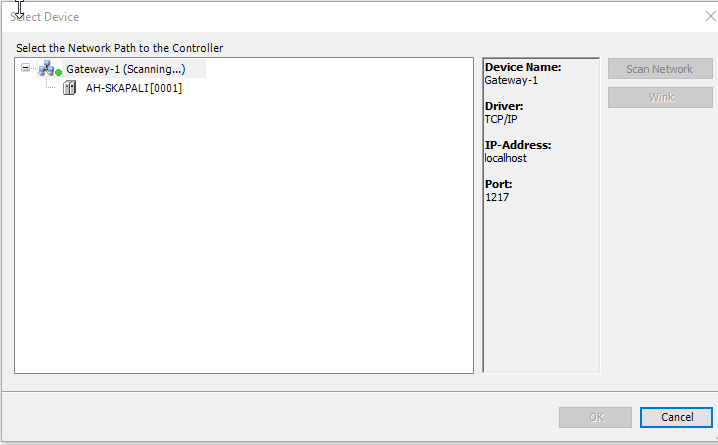5. Select Build > Build.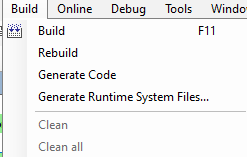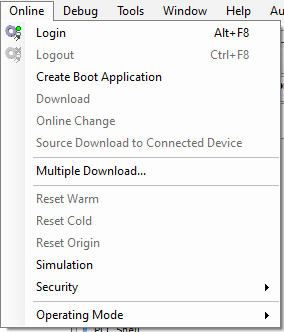7. Select Debug > Start.The soft PLC is now running the generated code.

Simulate the Model and Verify Generated Code

Simulate the Simulink ™ model and verify the generated code by comparing the simulation model results to the soft PLC results.The soft PLC results are retrieved by using the OPC UA connection.

1. Open the simple_cosim.slx file for the Simulink model.

% open_system('simple_cosim')

2. Simulate the model. Verify the generated code by comparing the model simulation results to the soft PLC cosimulation results.